## Change Flow Direction

In the Simple Gas Model tutorial, you created a simple open-loop gas model, which you then modified in the Change Flow Boundary Conditions tutorial. This example shows how to further modify this model, to investigate how the change in the gas flow direction affects the upstream temperature. To open the completed model, in the MATLAB® Command Window, type `ssc_gas_tutorial_step3`.

To investigate the change in the flow direction on the upstream temperature:

1. Open the model used in the Change Flow Boundary Conditions tutorial, by typing `ssc_gas_tutorial_step2`.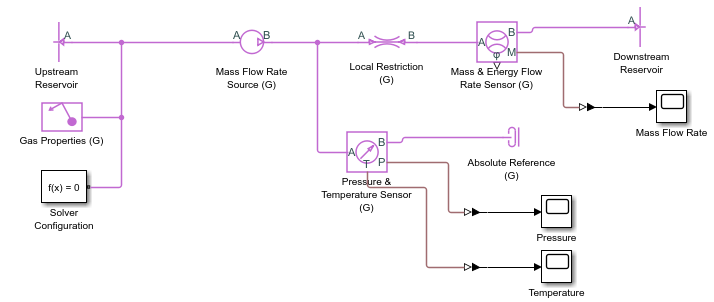2. Expose the input port of the Flow Rate Source (G) block by setting the Source type parameter to `Controlled`. Optionally, you can change the block name to Controlled Mass Flow Rate Source (G), for clarity.

3. Add a PS Sine Wave block and connect it to the input port of the Controlled Mass Flow Rate Source (G) block.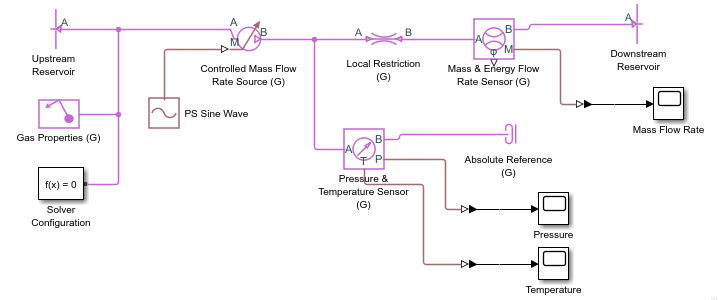4. Set the Amplitude parameter of the PS Sine Wave block to `0.15 kg/s` and Frequency specification to `Angular`.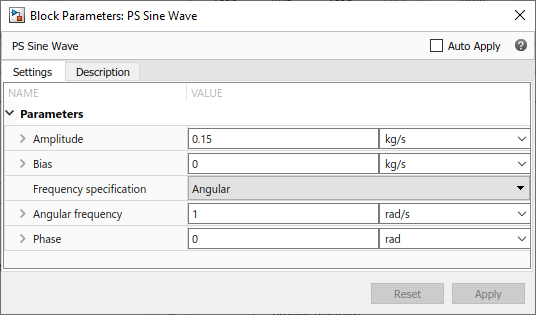5. Simulate the model. The mass flow rate through the restriction is now a sinusoidal wave with an amplitude of 0.15 kg/s.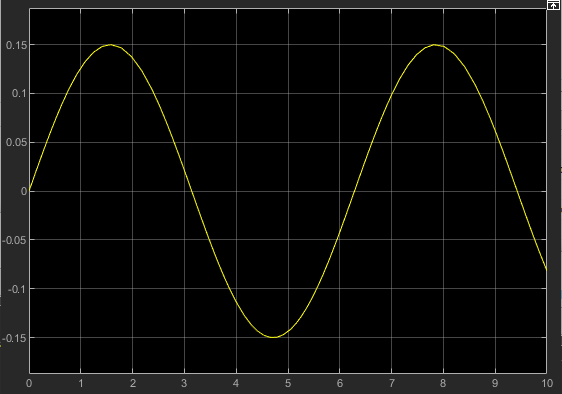Because the pressure on the right side of the restriction is fixed at atmospheric (as specified by the Downstream Reservoir block), the left side pressure (measured by the sensor) goes up and down, in order to produce the sinusoidal mass flow rate.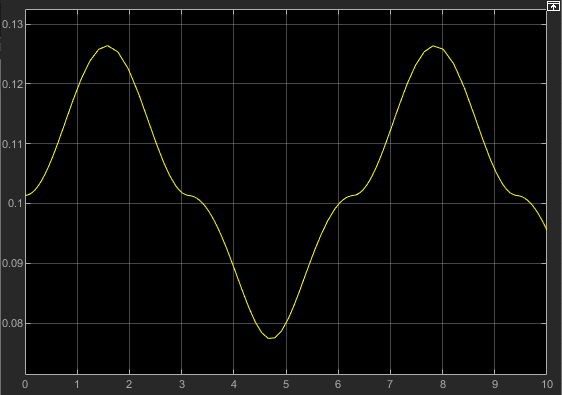The temperature measured by the sensor switches between 400 K and 293 K. This is because the sensor measures the temperature of the gas just upstream of the measured node. Therefore, when the mass flow rate is positive, the upstream temperature is 400 K (as specified by the Upstream Reservoir block). When the mass flow rate is negative, the upstream temperature is 293 K (as specified by the Downstream Reservoir block), because even though the local restriction does change the temperature of the gas flowing through it, in this model the change is minimal.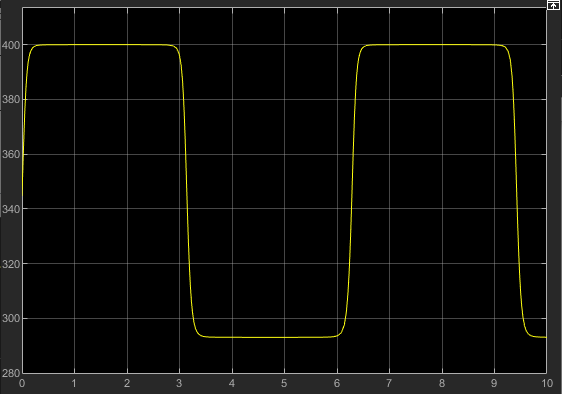6. As you can see, the switching between 400 K and 293 K is smoothed. This improves the simulation speed and robustness for flow reversal, that is, when the flow changes direction. The smoothing is controlled by the Mach number threshold for flow reversal parameter in the Gas Properties (G) block. Change this parameter value from `0.01` to `0.001` and rerun the simulation.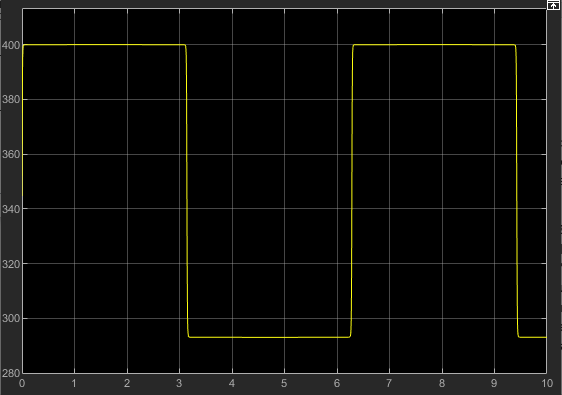The temperature now switches between 400 K and 293 K more sharply. Reducing the value of the Mach number threshold for flow reversal parameter can improve accuracy of the results, but may cause difficulties for the solver in a larger model, with large temperature variations and fast flow reversals.

7. Change the Mach number threshold for flow reversal parameter back to the default value of `0.01`, which provides sufficient accuracy for this model.

Because the temperature at a node represents the temperature of the gas just upstream of that node, other thermodynamic properties that depend on temperature also represent the gas just upstream of the node. For example, if you use the Thermodynamic Properties Sensor (G) block to measure gas density, the density value also goes up and down as the flow reverses, similar to the temperature plot.